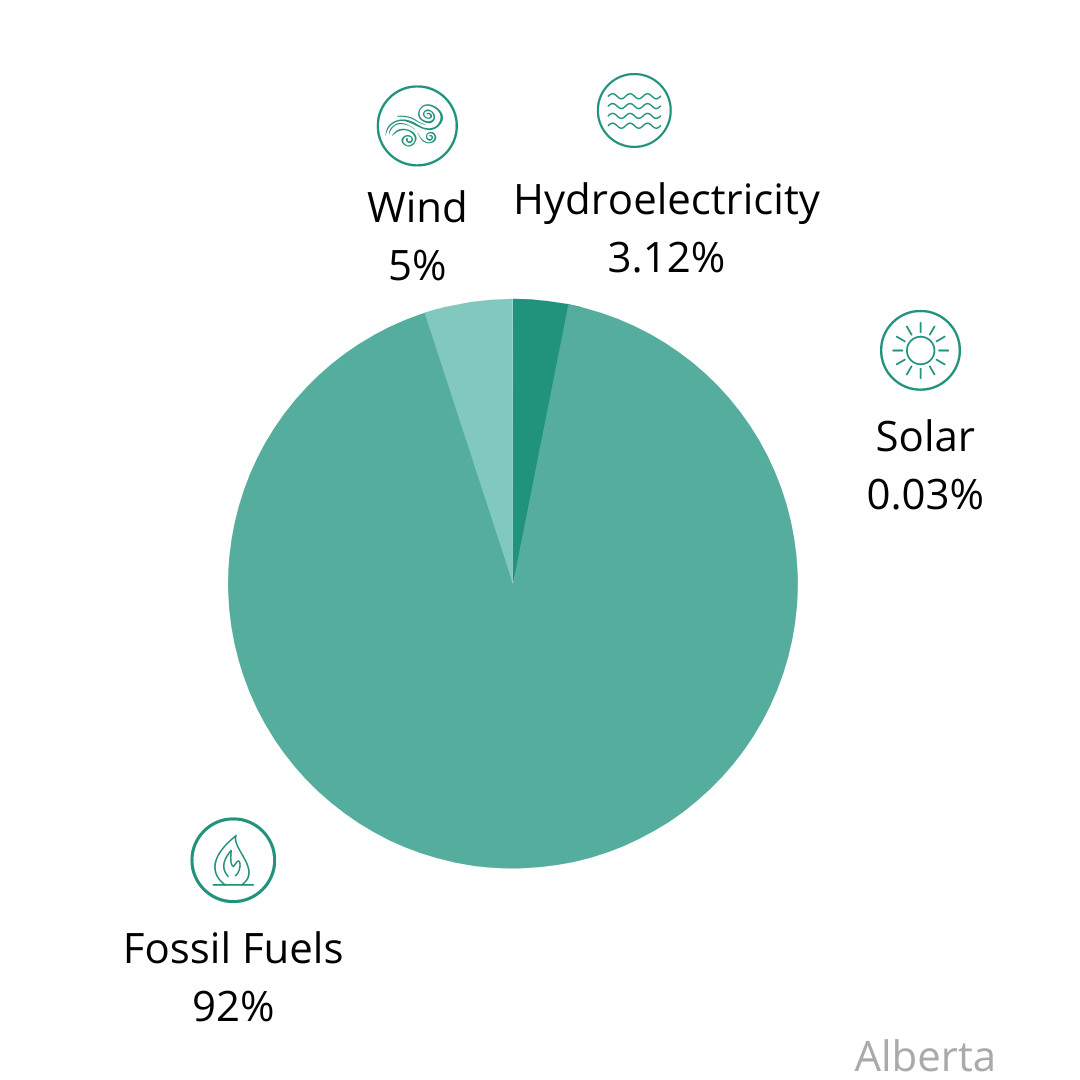# Electrical Energy Calculator - Alberta

This electrical energy calculator was created to support GreenLearning's Energy Revealed Program.

### Instructions

To use this calculator, input the watts of the device you are investigating and the minutes a day it is in use. Once you click on 'Calculate Energy' the electricity used (kWh), the greenhouse gases emissions (g of GHGs) produced and cost of electricity (\$) will be displayed. Keep scrolling to read more about out what these results mean.

### Kilowatt Hours (kWh)

A watt (W) is the unit used to measure the flow of electrical power. A kilowatt (kW) is 1,000 watts. A kilowatt hour (kWh) is a way to measure the amount of electricity used in an hour.

When you use electricity, the amount of energy consumed is measured in kWhs. The amount of electricity used (kWh) is dependent on how much power your device uses (W or kW) and how long you use it for (Minutes or Hours).

kWh Formula

kWh = (watts × hours)
___________
1,000

### Greenhouse Gases (GHGs)

GHGs refers to the collection of heat-trapping gases, including water vapour (H20), carbon dioxide (CO2), in our atmosphere. Generating electricity and using energy produces greenhouse gas emissions. Climate scientists agree that the main cause of the current climate crisis is the over-production of greenhouse gases by humans.

Some sources of electricity produce more GHGs than others. For example, burning fossil fuels, such as coal, oil and natural gas produces high amounts of GHGs compared to renewable energy sources, such as wind and solar energy.

Electricity Generation in AlbertaIn Alberta, electricity generates on average 620 grams of GHGs per kilowatt hour (620 g/kWh).

### Electricity Rates (\$)

Electricity rates are the cost you pay per kWh of energy used. In addition to paying for energy you use, providers will also charge you additional fees. For example, you might pay a delivery fee, which includes costs such as maintaining transmission lines, account management and billing and the energy lost on its way to you.
The average rate of electricity in Alberta is 9.56 cents per kWh (2021). Rates will differ for competitive retailers.